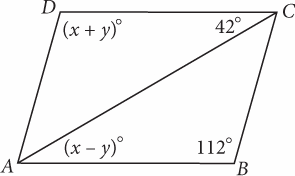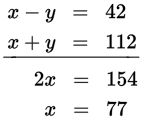# SAT Math Multiple Choice Question 959: Answer and Explanation

### Test Information

Question: 959

11.Note: Figure not drawn to scale.

Figure ABCD is a parallelogram. What is the product of xy?

• A. 2,695
• B. 2,940
• C. 4,704
• D. 6,468

Explanation:

A

Difficulty: Hard

Category: Additional Topics in Math / Geometry

Strategic Advice: Opposite angles of a parallelogram are congruent, and the diagonal acts as a transversal that is cutting two parallel lines (the opposite sides of the parallelogram).

Getting to the Answer: The alternate interior angles formed by the diagonal are congruent. This means x - y = 42 and x + y = 112. Set up a system of equations and solve it. The system is already arranged nicely to use elimination; combining the equations by adding them eliminates the y terms:Substitute this value into either of the original equations for x to find y: 77 + y = 112, so y = 35. The question asks for the product of x and y, so the correct answer is 77 × 35 = 2,695, which is (A).